# Selina Solutions Concise Maths Class 7 Chapter 1: Integers Exercise 1A

Selina Solutions Concise Maths Class 7 Chapter 1 Integers Exercise 1A covers important concepts like introduction, multiplication of integers, properties of multiplication and examples. Students can obtain a hold on these concepts by practising the textbook problems on a daily basis. Practicing problems one day before the exam will reduce their confidence in answering concept based questions. The solutions are prepared for the exercise wise problems to help students boost their exam preparation. For further details about the exercise problems, students can access Selina Solutions Concise Maths Class 7 Chapter 1 Integers Exercise 1A PDF, from the links which are provided here.

## Selina Solutions Concise Maths Class 7 Chapter 1: Integers Exercise 1A Download PDF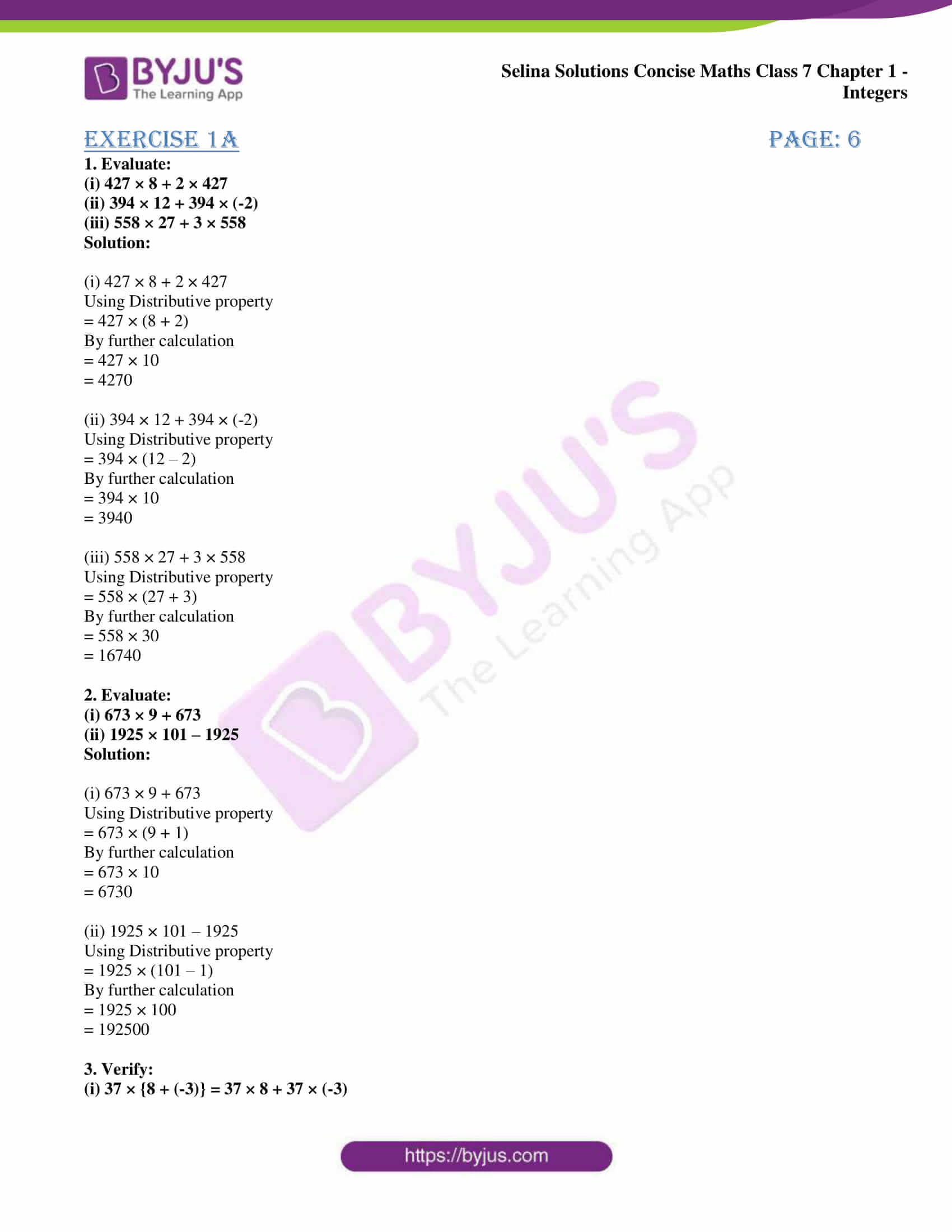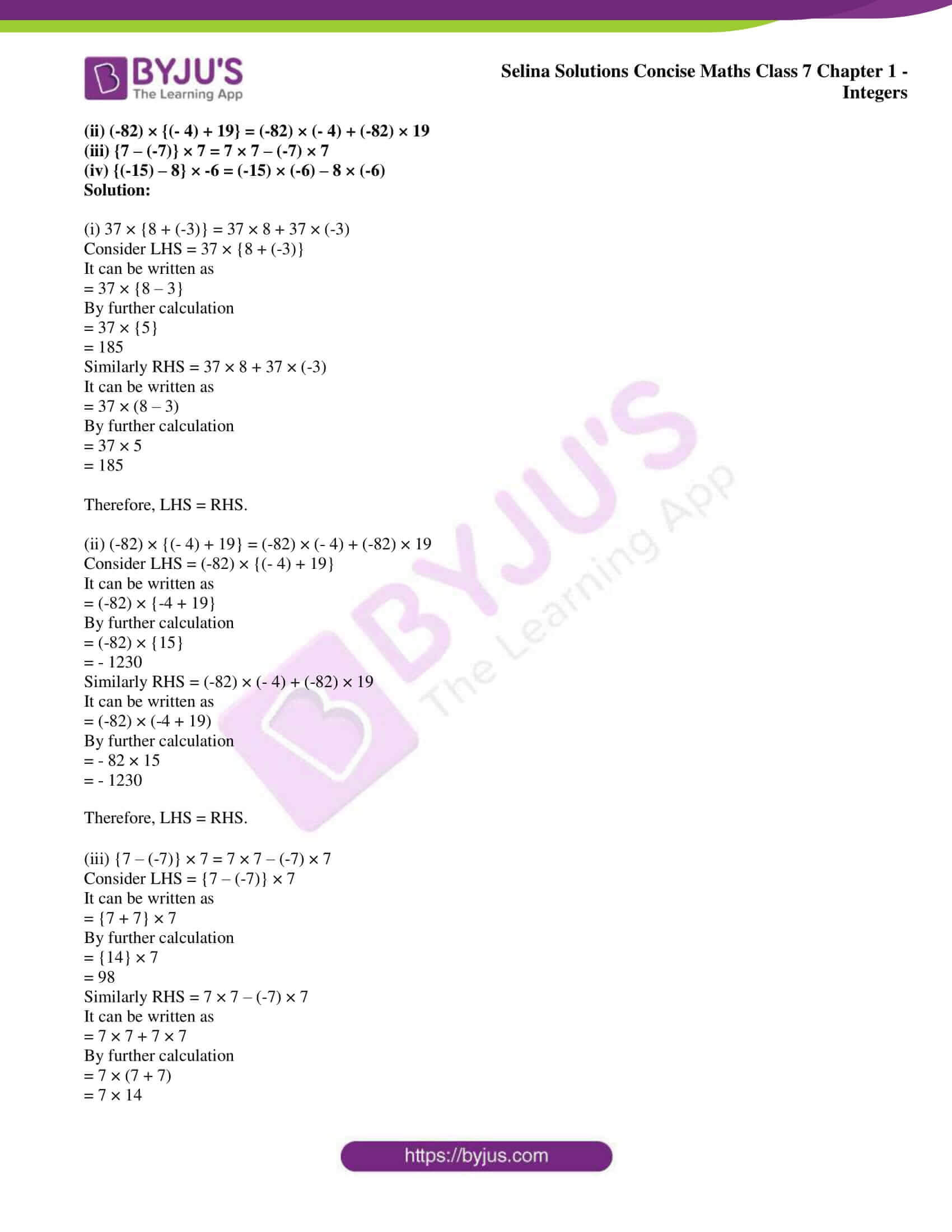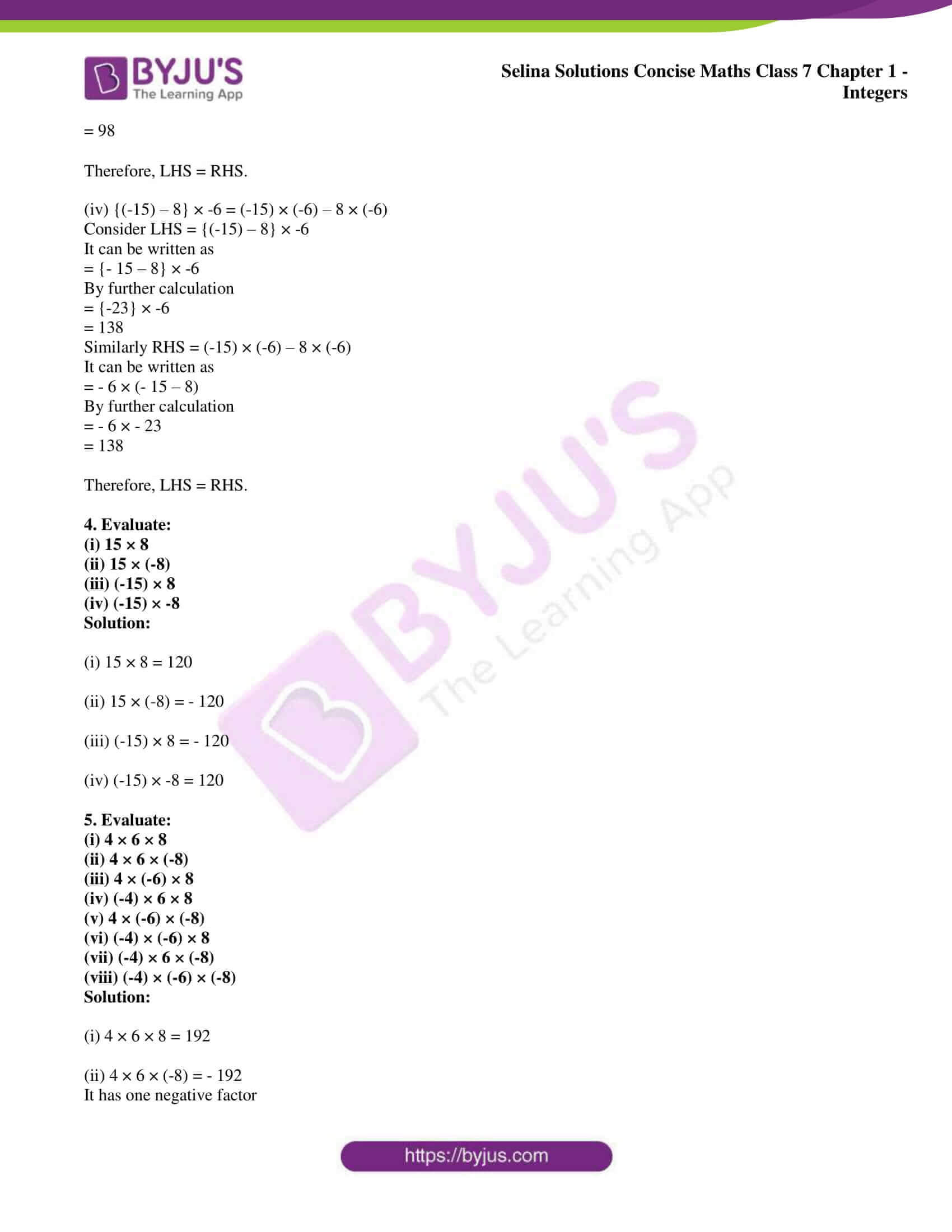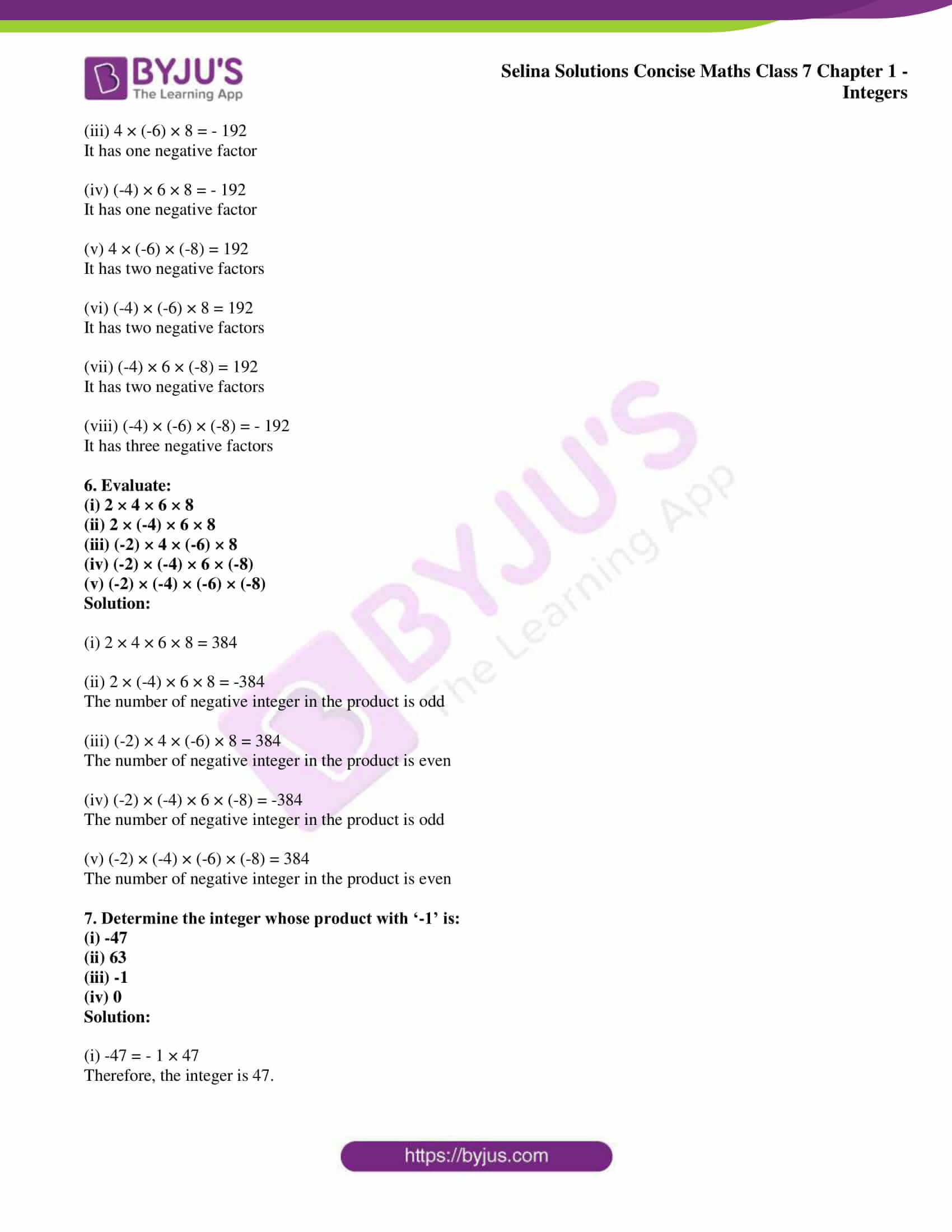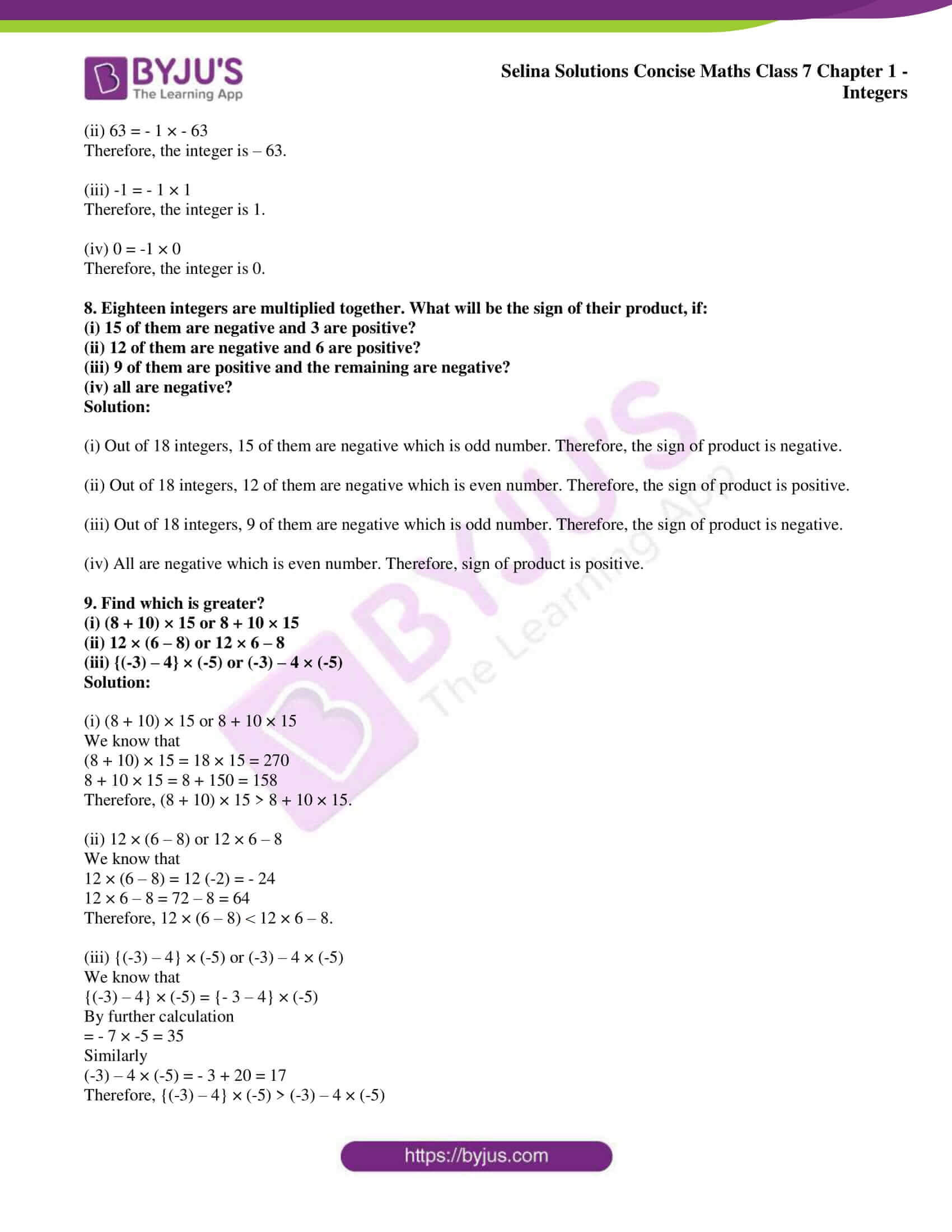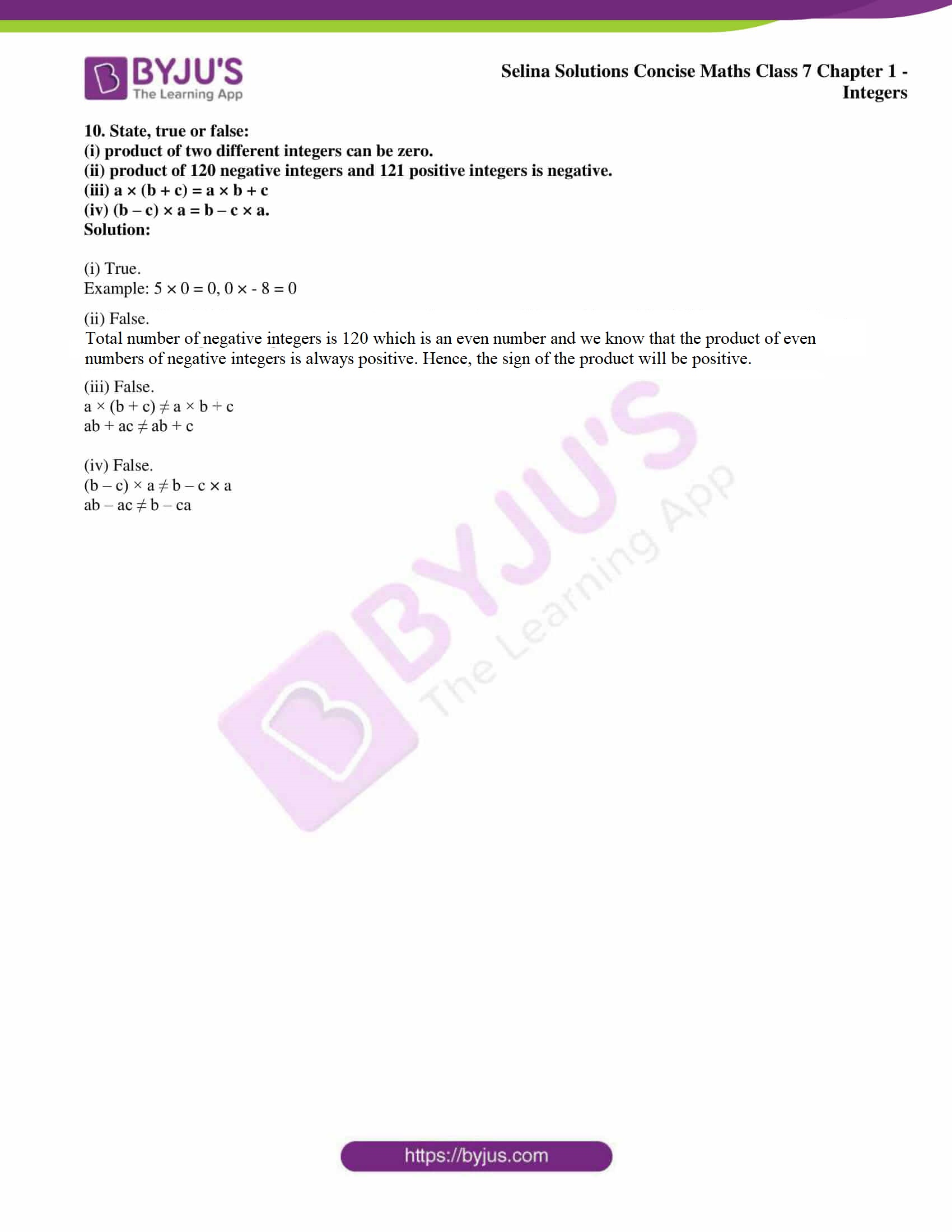### Access other exercises of Selina Solutions Concise Maths Class 7 Chapter 1: Integers

Exercise 1B Solutions

Exercise 1C Solutions

Exercise 1D Solutions

### Access Selina Solutions Concise Maths Class 7 Chapter 1: Integers Exercise 1A

1. Evaluate:

(i) 427 × 8 + 2 × 427

(ii) 394 × 12 + 394 × (-2)

(iii) 558 × 27 + 3 × 558

Solution:

(i) 427 × 8 + 2 × 427

Using Distributive property

= 427 × (8 + 2)

By further calculation

= 427 × 10

= 4270

(ii) 394 × 12 + 394 × (-2)

Using Distributive property

= 394 × (12 – 2)

By further calculation

= 394 × 10

= 3940

(iii) 558 × 27 + 3 × 558

Using Distributive property

= 558 × (27 + 3)

By further calculation

= 558 × 30

= 16740

2. Evaluate:

(i) 673 × 9 + 673

(ii) 1925 × 101 – 1925

Solution:

(i) 673 × 9 + 673

Using Distributive property

= 673 × (9 + 1)

By further calculation

= 673 × 10

= 6730

(ii) 1925 × 101 – 1925

Using Distributive property

= 1925 × (101 – 1)

By further calculation

= 1925 × 100

= 192500

3. Verify:

(i) 37 × {8 + (-3)} = 37 × 8 + 37 × (-3)

(ii) (-82) × {(- 4) + 19} = (-82) × (- 4) + (-82) × 19

(iii) {7 – (-7)} × 7 = 7 × 7 – (-7) × 7

(iv) {(-15) – 8} × -6 = (-15) × (-6) – 8 × (-6)

Solution:

(i) 37 × {8 + (-3)} = 37 × 8 + 37 × (-3)

Consider LHS = 37 × {8 + (-3)}

It can be written as

= 37 × {8 – 3}

By further calculation

= 37 × {5}

= 185

Similarly RHS = 37 × 8 + 37 × (-3)

It can be written as

= 37 × (8 – 3)

By further calculation

= 37 × 5

= 185

Therefore, LHS = RHS.

(ii) (-82) × {(- 4) + 19} = (-82) × (- 4) + (-82) × 19

Consider LHS = (-82) × {(- 4) + 19}

It can be written as

= (-82) × {-4 + 19}

By further calculation

= (-82) × {15}

= – 1230

Similarly RHS = (-82) × (- 4) + (-82) × 19

It can be written as

= (-82) × (-4 + 19)

By further calculation

= – 82 × 15

= – 1230

Therefore, LHS = RHS.

(iii) {7 – (-7)} × 7 = 7 × 7 – (-7) × 7

Consider LHS = {7 – (-7)} × 7

It can be written as

= {7 + 7} × 7

By further calculation

= {14} × 7

= 98

Similarly RHS = 7 × 7 – (-7) × 7

It can be written as

= 7 × 7 + 7 × 7

By further calculation

= 7 × (7 + 7)

= 7 × 14

= 98

Therefore, LHS = RHS.

(iv) {(-15) – 8} × -6 = (-15) × (-6) – 8 × (-6)

Consider LHS = {(-15) – 8} × -6

It can be written as

= {- 15 – 8} × -6

By further calculation

= {-23} × -6

= 138

Similarly RHS = (-15) × (-6) – 8 × (-6)

It can be written as

= – 6 × (- 15 – 8)

By further calculation

= – 6 × – 23

= 138

Therefore, LHS = RHS.

4. Evaluate:

(i) 15 × 8

(ii) 15 × (-8)

(iii) (-15) × 8

(iv) (-15) × -8

Solution:

(i) 15 × 8 = 120

(ii) 15 × (-8) = – 120

(iii) (-15) × 8 = – 120

(iv) (-15) × -8 = 120

5. Evaluate:

(i) 4 × 6 × 8

(ii) 4 × 6 × (-8)

(iii) 4 × (-6) × 8

(iv) (-4) × 6 × 8

(v) 4 × (-6) × (-8)

(vi) (-4) × (-6) × 8

(vii) (-4) × 6 × (-8)

(viii) (-4) × (-6) × (-8)

Solution:

(i) 4 × 6 × 8 = 192

(ii) 4 × 6 × (-8) = – 192

It has one negative factor

(iii) 4 × (-6) × 8 = – 192

It has one negative factor

(iv) (-4) × 6 × 8 = – 192

It has one negative factor

(v) 4 × (-6) × (-8) = 192

It has two negative factors

(vi) (-4) × (-6) × 8 = 192

It has two negative factors

(vii) (-4) × 6 × (-8) = 192

It has two negative factors

(viii) (-4) × (-6) × (-8) = – 192

It has three negative factors

6. Evaluate:

(i) 2 × 4 × 6 × 8

(ii) 2 × (-4) × 6 × 8

(iii) (-2) × 4 × (-6) × 8

(iv) (-2) × (-4) × 6 × (-8)

(v) (-2) × (-4) × (-6) × (-8)

Solution:

(i) 2 × 4 × 6 × 8 = 384

(ii) 2 × (-4) × 6 × 8 = -384

The number of negative integer in the product is odd

(iii) (-2) × 4 × (-6) × 8 = 384

The number of negative integer in the product is even

(iv) (-2) × (-4) × 6 × (-8) = -384

The number of negative integer in the product is odd

(v) (-2) × (-4) × (-6) × (-8) = 384

The number of negative integer in the product is even

7. Determine the integer whose product with ‘-1’ is:

(i) -47

(ii) 63

(iii) -1

(iv) 0

Solution:

(i) -47 = – 1 × 47

Therefore, the integer is 47.

(ii) 63 = – 1 × – 63

Therefore, the integer is – 63.

(iii) -1 = – 1 × 1

Therefore, the integer is 1.

(iv) 0 = -1 × 0

Therefore, the integer is 0.

8. Eighteen integers are multiplied together. What will be the sign of their product, if:

(i) 15 of them are negative and 3 are positive?

(ii) 12 of them are negative and 6 are positive?

(iii) 9 of them are positive and the remaining are negative?

(iv) all are negative?

Solution:

(i) Out of 18 integers, 15 of them are negative which is odd number. Therefore, the sign of product is negative.

(ii) Out of 18 integers, 12 of them are negative which is even number. Therefore, the sign of product is positive.

(iii) Out of 18 integers, 9 of them are negative which is odd number. Therefore, the sign of product is negative.

(iv) All are negative which is even number. Therefore, sign of product is positive.

9. Find which is greater?

(i) (8 + 10) × 15 or 8 + 10 × 15

(ii) 12 × (6 – 8) or 12 × 6 – 8

(iii) {(-3) – 4} × (-5) or (-3) – 4 × (-5)

Solution:

(i) (8 + 10) × 15 or 8 + 10 × 15

We know that

(8 + 10) × 15 = 18 × 15 = 270

8 + 10 × 15 = 8 + 150 = 158

Therefore, (8 + 10) × 15 > 8 + 10 × 15.

(ii) 12 × (6 – 8) or 12 × 6 – 8

We know that

12 × (6 – 8) = 12 (-2) = – 24

12 × 6 – 8 = 72 – 8 = 64

Therefore, 12 × (6 – 8) < 12 × 6 – 8.

(iii) {(-3) – 4} × (-5) or (-3) – 4 × (-5)

We know that

{(-3) – 4} × (-5) = {- 3 – 4} × (-5)

By further calculation

= – 7 × -5 = 35

Similarly

(-3) – 4 × (-5) = – 3 + 20 = 17

Therefore, {(-3) – 4} × (-5) > (-3) – 4 × (-5)

10. State, true or false:

(i) product of two different integers can be zero.

(ii) product of 120 negative integers and 121 positive integers is negative.

(iii) a × (b + c) = a × b + c

(iv) (b – c) × a = b – c × a.

Solution:

(i) True.

Example: 5 × 0 = 0, 0 × – 8 = 0

(ii) False.

The total number of negative integers is 120 which is an even number and we know that the product of even numbers of negative integers is always positive. Hence, the sign of the product will be positive.

(iii) False.

a × (b + c) ≠ a × b + c

ab + ac ≠ ab + c

(iv) False.

(b – c) × a ≠ b – c × a

ab – ac ≠ b – ca